Ex 6.3

Chapter 6 Class 11 Linear Inequalities
Serial order wise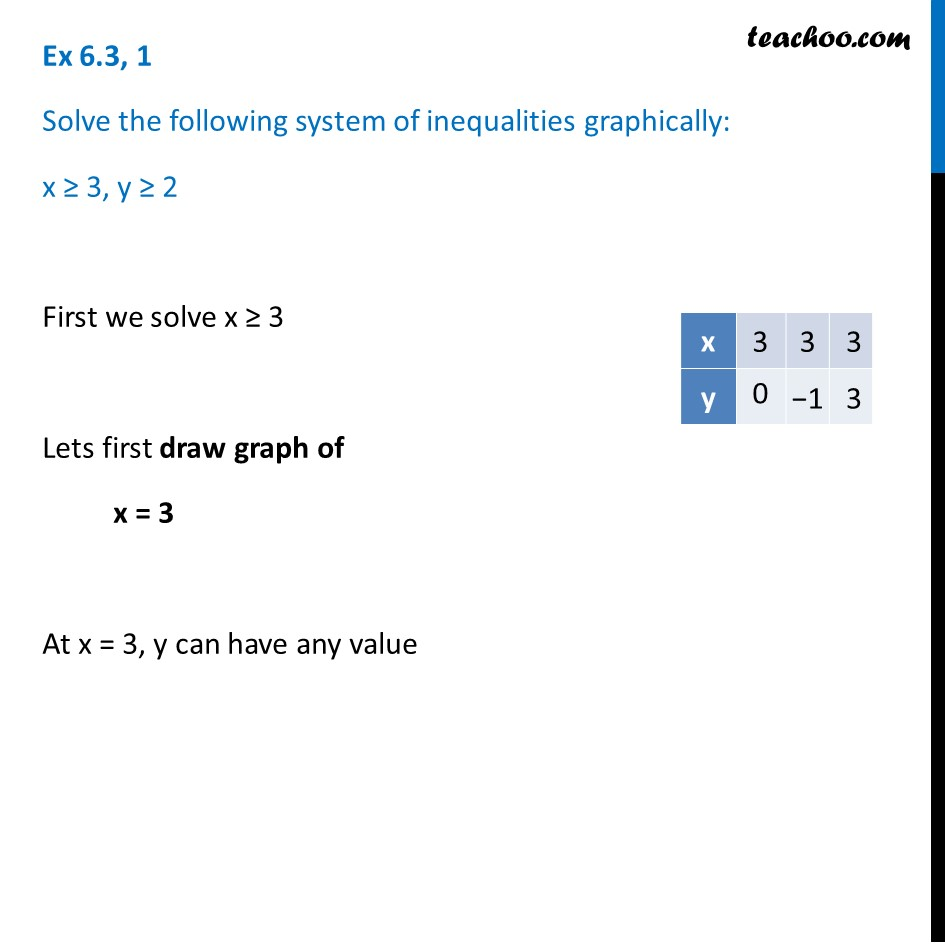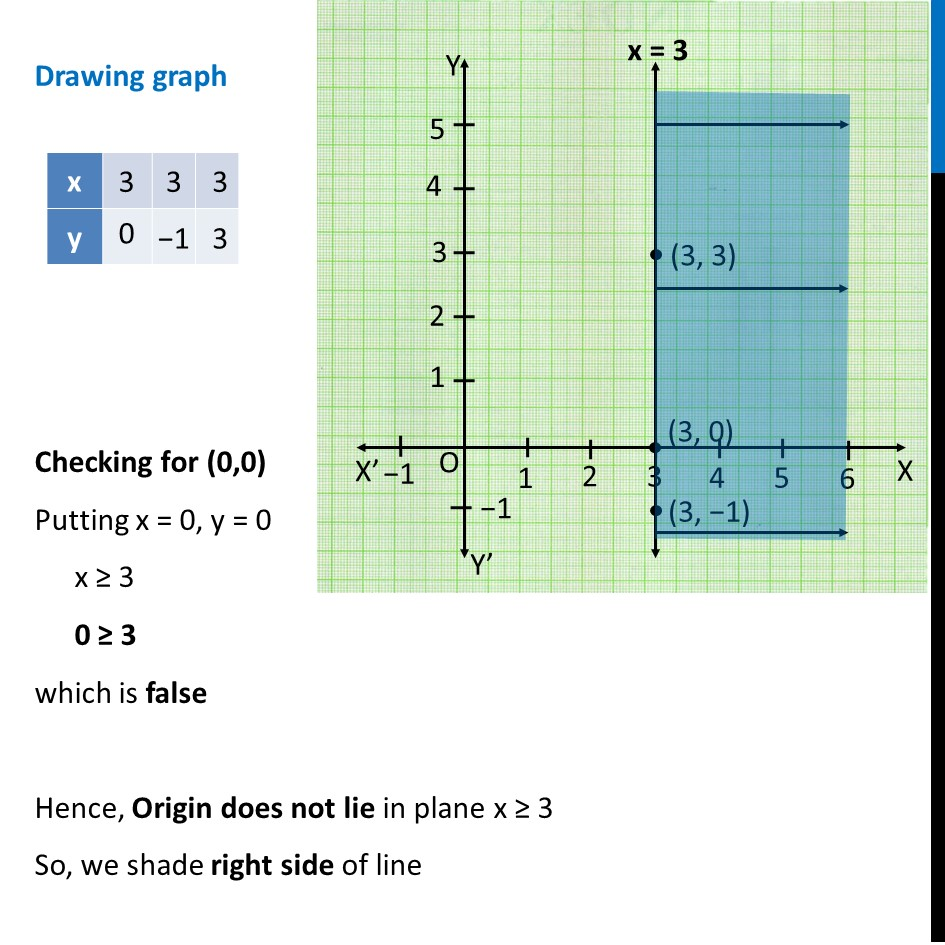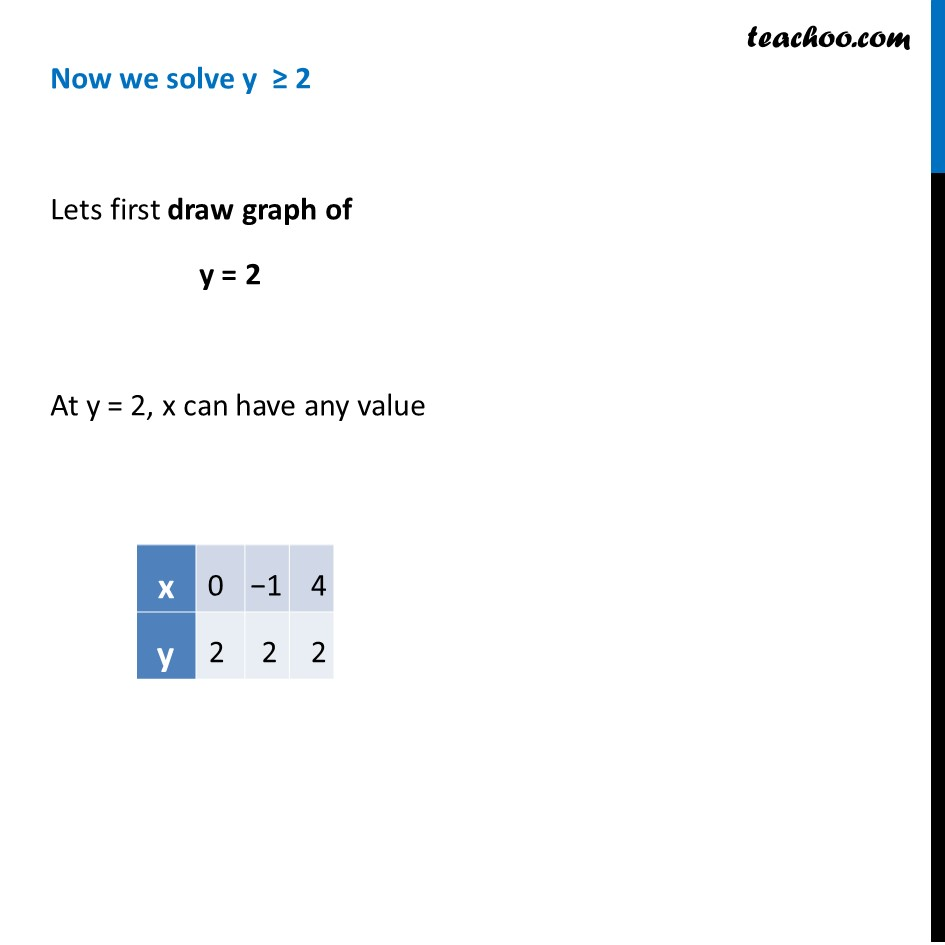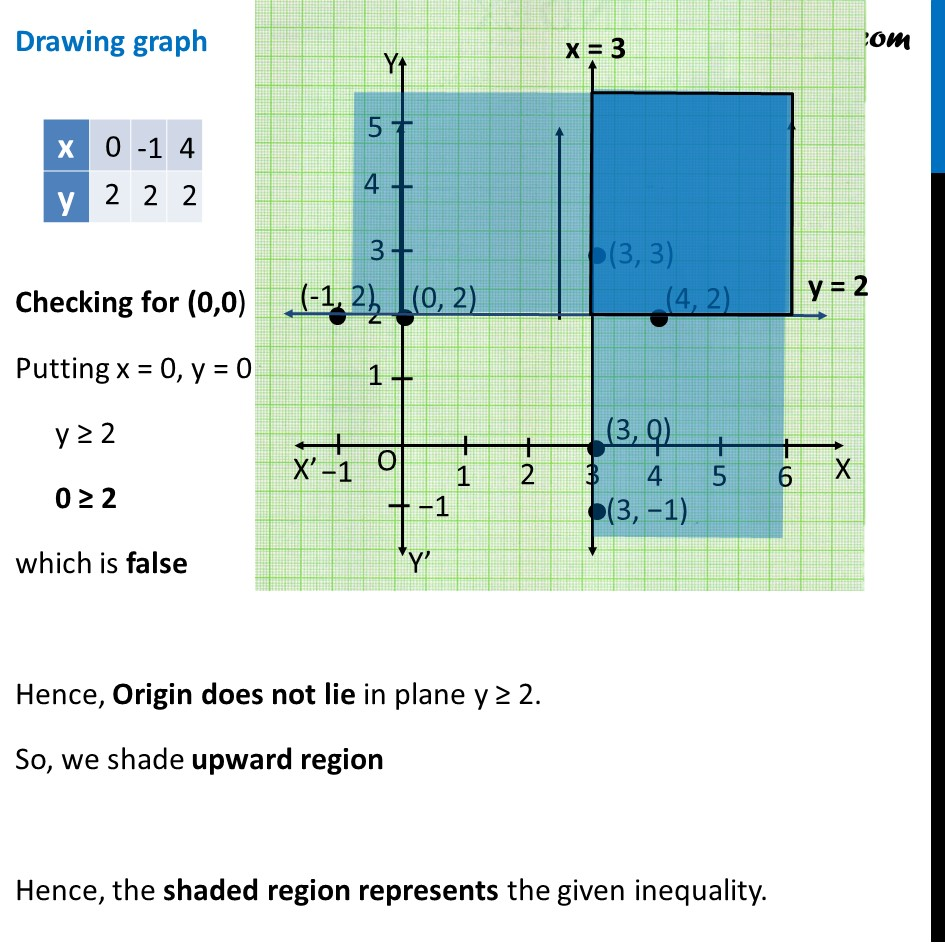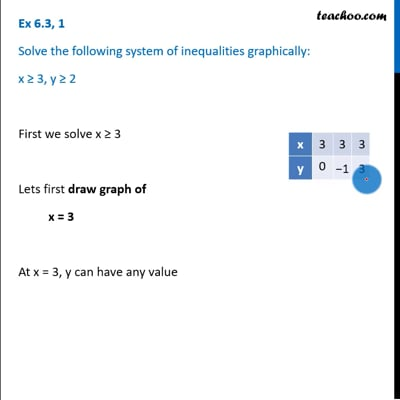This video is only available for Teachoo black users

Solve all your doubts with Teachoo Black (new monthly pack available now!)

### Transcript

Ex 6.3, 1 Solve the following system of inequalities graphically: x ≥ 3, y ≥ 2 First we solve x ≥ 3 Lets first draw graph of x = 3 At x = 3, y can have any value Drawing graph Checking for (0,0) Putting x = 0, y = 0 x ≥ 3 0 ≥ 3 which is false Hence, Origin does not lie in plane x ≥ 3 So, we shade right side of line Now we solve y ≥ 2 Lets first draw graph of y = 2 At y = 2, x can have any value Drawing graph Checking for (0,0) Putting x = 0, y = 0 y ≥ 2 0 ≥ 2 which is false Hence, Origin does not lie in plane y ≥ 2. So, we shade upward region Hence, the shaded region represents the given inequality.